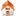# Spherical Slepian functions and the polar gap in geodesy

## Frederik J Simons1 and F. A. Dahlen2

### 2 Geosciences Department Princeton University Princeton NJ 08544, USA

Geoph. J. Int., 2006, 166 (3), 1039-1061, doi:10.1111/j.1365-246X.2006.03065.x
 Reprint Related work Software Publications

#### Figures

1. Figure 01 Sketch illustrating the geometry of the spherical concentration problem to the single and double polar cap.
2. Figure 02 Spatial eigensolutions to the problem of concentrating a bandlimited spherical harmonic expansion to the latitudinal belt.
3. Figure 03 Spatial eigensolutions to the problem of concentrating a bandlimited spherical harmonic expansion to the double polar cap.
4. Figure 04 First four of Grünbaum's eigenfunctions on the belt with L=18 and a double polar cap of radius 30°.
5. Figure 05 Last four of Grünbaum's eigenfunctions on the belt with L=18 and a double polar cap of radius 30°.
6. Figure 06 Cumulative energy of the latitudinal belt tapers in the space-domain
7. Figure 07 Cumulative energy of the double polar cap tapers in the space-domain
8. Figure 08 Ranked eigenvalues of the concentration to a latitudinal belt for a fixed bandwidth.
9. Figure 09 Ranked Grünbaum eigenvalues for L=18 and different sizes of the double polar cap.
10. Figure 10 Average error-to-signal ratio for TH=10 and L=45 for different signal-to-noise ratios and spherical harmonic damping levels.
11. Figure 11 Error-to-signal ratio for TH=10 and L=45 for different signal-to-noise ratios and spherical harmonic damping levels.
12. Figure 12 Average error-to-signal ratio for TH=10 and L=45 for different signal-to-noise ratios and Slepian truncation levels.
13. Figure 13 Error-to-signal ratio for TH=10 and L=45 for different signal-to-noise ratios and Slepian truncation levels.
14. Figure 14 Breakdown of mse, var, and bias, as a function of damping/truncation level and, for the optimum values, as a function of colatitude.

1. Figure X1 Spectral eigensolutions to the problem of concentrating a spacelimited function within a spectral band. (Not included in paper.)
2. Figure X2 Eigenfunctions of the double circular polar cap of radius 40° for a fixed bandwidth of L= 18 in decreasing order of concentration. (Not included in paper.)
3. Figure X3 Up- and downward continuation of spherical Slepian functions. (Not included in paper.)

Frederik Simons